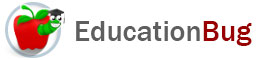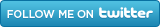PUBLIC SCHOOLS PRIVATE SCHOOLS SCHOOL DISTRICTS COLLEGES PUBLIC LIBRARIES JOBS BLOG RESOURCES
Career Education
Education Choices
Education Costs
Education History and General Information
Education Issues
Education Resources
Family Involvement in Education
Students w Special Needs
Subject Information and Homework Help
Teaching and Learning
Testing
By Types of Schools
By Specific Audiences
GeneralInequalities

What is an inequality, what different types of inequalities exist, and how can you use them? Learn the answers to these questions about inequalities and more in this article.

Because we often think in dualisms in math - addition and subtraction; multiplication and division; positive and negative numbers - itâ€™s possible that some people might look at the term inequalities and knowing about equalities - come up with a too narrow view of what the term means. This article clarifies the meaning of inequalities in mathematics.

Equalities - a Jumping Off Point

Seen one of these recently: = ? Itâ€™s an equal sign and we use them very often, in school, at work, and in doing figuring around the house. They are the sign that we are working on an equality, what we more often call an equation. An equation is simply a written way of expressing the equality of two expressions.

So whatâ€™s an inequality? Well, thereâ€™s more than one answer to that. So letâ€™s examine the various things that are not equalities.

Types of Inequalities

There are a number of types of inequality, and seven types that are frequently used and are associated with symbols that you should know:

Â¹ means â€œnot equal to,â€ so a Â¹ b means â€œa does not equal b.â€

Â»  means â€œalmost equal to,â€ so a Â»  b means â€œa is almost equal to b.â€

< means â€œless than,â€ so a < b means â€œa is less than b.â€

> means â€œgreater than,â€ so a > b means â€œa is greater than b.â€

â‰¤ means â€œless than or equal to,â€ so a â‰¤ b means â€œa is less than or equal to b.â€

Â³ means â€œgreater than or equal to,â€ so Â³  b means â€œa is greater than or equal to b.â€

@ means â€œapproximately equal to,â€ so a @ b means â€œa is approximately equal to b.â€

Inequalities can also express multiple relationships at once. Here are some examples:

0 < a > 10 means that â€œa is a number between 0 and 10.â€

0 â‰¤ a Â³ 10 means that â€œa is a number in the range 0 to 10â€ (note the distinction from the inequality above).

a < b < c means that â€œa is less than b, which in turn is less than c.â€

What Inequalities Tell Us

Equalities are, by definition, rather definitive. They say, with more or less precision, what something is. Inequalities usually leave a lot more possibilities open than equalities, but they often help narrow the range of possibilities. For example, if we were to find a problem that told us that x is a negative whole number and x > -3, then weâ€™ve narrowed the choices to -2 and -1.

In some cases, however, inequalities can provide enough information to gain an exact or precise answer. Hereâ€™s an example of a problem that includes an equation and an inequality as its parameters:

x2 = 4

x < 0

By itself, x2 = 4 does not have a single answer. x could either be 2 or -2. The inequality allows us to pinpoint the answer: because we know that x is less than zero, it follows that x = -2

Hereâ€™s another example:

Let x and y be positive integers (whole numbers):

x + y = 7

x < 2 < y

Since the only positive whole number less than 2 is one, x = 1 and so y must be 6.

How to Use Inequalities

Inequalities have similar properties to equalities in that a similar operation may be performed on both sides of the inequality to create another true inequality. This means one can:

• add the same quantity to both sides of an inequality
• subtract the same quantity from both sides of an inequality
• multiply the same quantity by both sides of an inequality
• divide both sides of an inequality by the same, nonzero quantity
• in most cases, apply the same function to both sides of an inequality